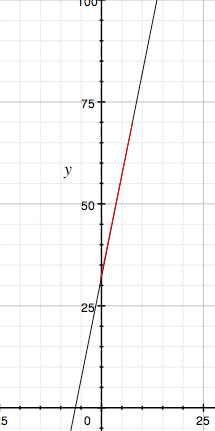SEARCH HOMEMath Central Quandaries & QueriesQuestion from Amanda, a student: I'm studying for the mid-term and I can't seem to grasp this one question on my review sheet. Can you help me? Find the range of a function y = 32 + 5x where 0 < x < 8 and x is an integer.Hi Amanda,

The graph of y = 32 + 5x is a straight line with slope 5, that is it slopes upwards to the right. When x = 0 you get y = 32 and when x = 8 you get y = 72. Since you know the graph is a line segment from (9,32) to (8,72) the range is 32 < y < 72.y = 32 + 5x

PennyMath Central is supported by the University of Regina and The Pacific Institute for the Mathematical Sciences.